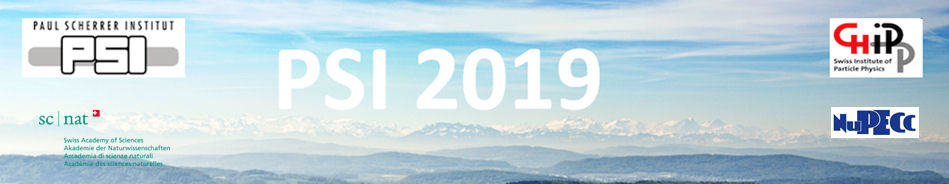#Physics of fundamental Symmetries and Interactions - PSI2019

Oct 20 – 25, 2019
PSI
Europe/Zurich timezone

## Testing lepton flavor universality with pion decays

Oct 23, 2019, 9:30 AM
30m
WHGA/001 - Auditorium (PSI)

Invited Talk

### Speaker

Prof. Douglas Bryman (University of British Columbia and TRIUMF)

### Description

Since the discovery of the muon, the lack of understanding of flavor is one of the most intriguing and persistent problems in particle physics. Many hypotheses which extend the Standard Model suggest that observations of non-universal charged current interactions could be due to effects at high mass scales, possibly approaching 1000 TeV, or to the existence of sterile neutrinos. Measurement of the pion decay branching ratio $R_{\pi\rightarrow e}=\frac{\Gamma(\pi^+\to e^+\nu +\pi^+\to e^+\nu\gamma)}{ \Gamma(\pi^+\to \mu^+\nu +\pi^+\to \mu^+\nu\gamma)}$ in comparison with the extraordinarily precise Standard Model prediction provides one of the best tests of universality, currently indicating consistency with $e-\mu$ universality at the 0.1 % level. This presentation will discuss the PIENU experiment at TRIUMF which aims to improve the precision of the measurement of $R_{\pi\to e}$ and to search for evidence of sterile neutrinos involved in pion decay.

### Primary authors

Prof. Douglas Bryman (University of British Columbia and TRIUMF)

### Presentation materials

 BrymanPSI2019.pdf BrymanPSI2019.ppt